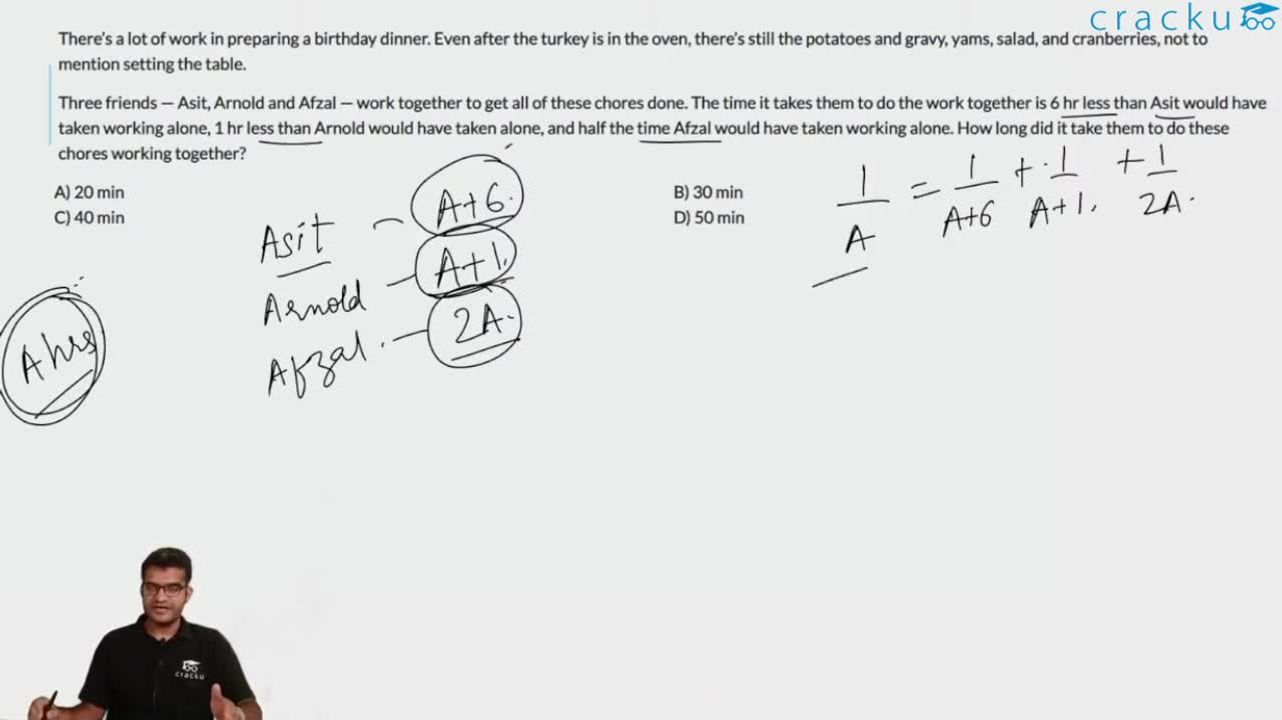Question 32

# There’s a lot of work in preparing a birthday dinner. Even after the turkey is in the oven, there’s still the potatoes and gravy, yams, salad, and cranberries, not to mention setting the table.Three friends — Asit, Arnold and Afzal — work together to get all of these chores done. The time it takes them to do the work together is 6 hr less than Asit would have taken working alone, 1 hr less than Arnold would have taken alone, and half the time Afzal would have taken working alone. How long did it take them to do these chores working together?

Solution

Let the time taken working together be t.
Time taken by Arnold = t+1
Time taken by Asit = t+6
Time taken by Afzal = 2t
Work done by each person in one day = $$\frac{1}{(t+1)}+\frac{1}{(t+6)}+\frac{1}{2t}$$
Total portion of workdone in one day $$=\frac{1}{t}$$
$$\frac{1}{(t+1)}+\frac{1}{(t+6)}+\frac{1}{2t}=\frac{1}{t}$$
$$\frac{1}{(t+1)}+\frac{1}{(t+6)}=\frac{2-1}{2t}$$
$$2t+7=\frac{(t+1)\cdot(t+6)}{2t}$$
$$3t^2-7t+6=0 \longrightarrow\ t=\frac{2}{3}$$or $$t=-3$$
Therefore total time = $$\frac{2}{3}$$hours = 40mins

Alternatively,
$$\frac{1}{(t+1)}+\frac{1}{(t+6)}+\frac{1}{2t}=\frac{1}{t}$$
From the options, if time $$= 40$$ min, that is, $$t = \frac{2}{3}$$
LHS = $$\frac{3}{5} + \frac{3}{20} + \frac{3}{4} = \frac{(12+3+15)}{20} = \frac{30}{20} = \frac{3}{2}$$
RHS = $$\frac{1}{t}=\frac{3}{2}$$
The equation is satisfied only in case of option C
Hence, C is correct

### View Video Solution